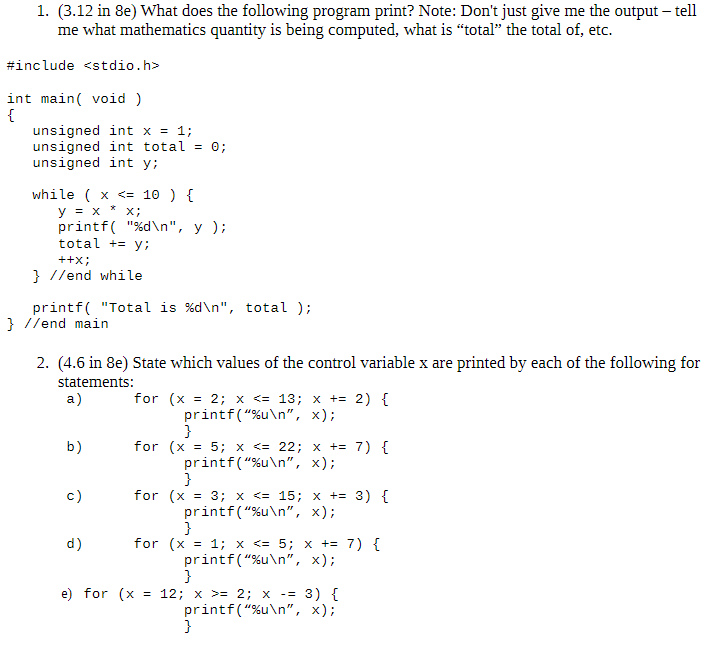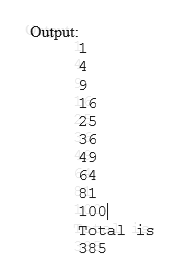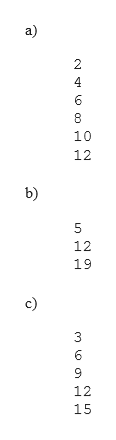1. (3.12 in 8e) What does the following program print? Note: Don't just give me the output - tellme what mathematics quantity is being computed, what is "total" the total of, etc.#include int main void){unsigned int x = 1;unsigned int totalunsigned int ywhile x

Questionhelp_outlineImage Transcriptionclose1. (3.12 in 8e) What does the following program print? Note: Don't just give me the output - tell me what mathematics quantity is being computed, what is "total" the total of, etc. #include int main void) { unsigned int x = 1; unsigned int total unsigned int y while x <= 10 ) [ у3х printf "%d\n", y ); total y х; ++x //end while printf( "Total is %d\n", total ); } //end main 2. (4.6 in 8e) State which values of the control variable x are printed by each of the following for statements: a) for (x 2; x <= 13; x += 2) printf("%u\n", x); } for (x 5; x <= 22; x += 7) { b) printf("%u\n", x); } c) for (x 3; x <= 15; x += 3) { printf("%u\n", x); } d) for (x 1; x <= 5; x += 7) printf("%u\n", x); } 12; x 2; x printf("%u\n", x); } e) for (x 3) fullscreen
Step 1

1)

The given code snippet is used to print the squares of numbers from 1 to 10. It calculates the sum of squares of numbers from 1 to 10 and print it on the screen. So the squares of numbers will be 1, 4, 9, 16, 36, 49, 64, 81, 100. The sum of squares is 385.help_outlineImage TranscriptioncloseOutput 1 4 9 16 25 36 49 64 81 100 Total is 385 fullscreen
Step 2

2) Output for the given statements:

Value of control variable "...help_outlineImage Transcriptionclosea) 6 10 12 b) 12 19 c) 3 6 9 12 15 CN N LO NLn fullscreen

Want to see the full answer?

See Solution

Want to see this answer and more?

Our solutions are written by experts, many with advanced degrees, and available 24/7

See Solution
Tagged in

Programing Language